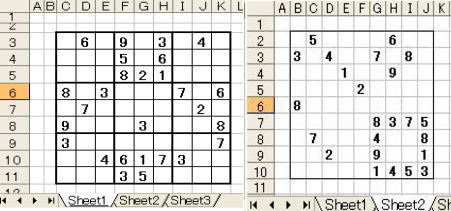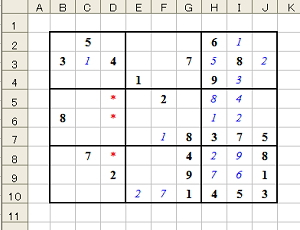### Sudoku PuzzleHere is an example solving Sudoku puzzle having three different types of 9 X 9, 16 X 16, and 25 X 25 cells with the use of Excel VBA program. Japanese

Figure 1.The range of puzzle must be enclosed with solid line and the starting cell must be set within a region of "A1:H8" cells as demonstrated in Figure 1.

Algorithm of solving game, for instance 9×9 cells, is as follow. At first, all vacant cells are filled with a string "abcdefghi", in which "a" and "b" … corresponds to 1, 2 and so. Several alphabetical characters are deleted according to a rule of Sudoku game. Thus, cells having only one letter can be altered to numerical figure.

Cells are divided into １ 〜 27 groups, i.e. Grp(1) to Grp(9) are Column, Grp(10) to Grp(18) are Row, and Grp(19) to Grp(27) are Block groups, respectively. Grp(0) is regarded as total cells, i.e. Range("C3:K11") in Figure 1, left.

Group number of a specified cell can be determined using private Function, i.e. cG(c), rG(c), bG(c). For example, if c is E8 cell, cG(c), rG(c), and bG(c) returns 3, 15, and 22.

WorksheetFunction.CountIf is particularly convenient in counting number of cells existing in each group. If WorksheetFunction.CountIf((Grp(10), "*c*")) equals to 2, number of cells containing "c" character in the range of "C3:K3" is only two. If this Function measures 1, the cell can be numerically fixed (i).

Figure 2．9×9 cells
 5 6 3 4 7 8 1 9 2 8 8 3 7 5 7 4 8 2 9 1 1 4 5 3

(1) If a Column group (or a Row group) has only two cells containing some character and relevant two cells are both in the same Block group, other cells in the Block cannot contain this character(!). Furthermore, If a Block group has only two cells containing some character and the two cells are both in same Column group (or same Row group), other cells in the Column group (or Row group) cannot contain this character (!).

(2) If a group has only two cells containing both "c" and "e" characters for instance, and the two cells are both identical cells, the relevant two cells could be fixed as "ce"(*). The same manner may be applied for three characters in three cells(^).

In the case of upper-graded puzzle beyond scheme described above or originally impossible one, "Give UP" message will appear. If successfully dissolved, message such as "iiii!!!!!^iiiiii-3.97" is shown indicating history of successful methods and time needed for solving.

Figure 3．16×16 cells
 2 9 8 3 8 5 2 11 16 1 7 4 3 5 11 6 4 13 12 7 2 1 7 16 12 8 15 13 11 10 4 7 7 9 10 5 13 12 14 4 3 1 15 5 11 15 1 3 14 9 16 13 7 11 5 2 1 3 4 10 12 8 15 16 7 16 15 5 2 3 15 7 11 5 7 12 16 8 2 1 9 10 16 13 14 12 9 1

```

Dim c0 As Range, Mas, sM, success As Boolean, Grp() As Range
Sub sudoku()
Dim cel(9) As Range                      '---[ Sudoku  Ver 5.1 ]---
Call SelSt(w):  Set c0 = Range(w)
For i = 1 To 25:     If c0.Cells(i + 1, 1).Borders(7).LineStyle <> 1 Then Exit For
Next:  Mas = i:             If Mas = 16 Or Mas = 25 Then Else Mas = 9
ReDim Grp(Mas * 3), nG(1 To Mas, 1 To Mas * 3)
Set Grp(0) = Range(c0, c0.Cells(Mas, Mas)):    sM = Sqr(Mas)
If WorksheetFunction.CountIf(Grp(0), ">0") = 0 Then Grp(0).Select: MsgBox "Range missing":: End
For i = 1 To Mas:   Set Grp(i) = Grp(0).Columns(i).Cells:     Set Grp(Mas + i) = Grp(0).Rows(i).Cells:
Set Grp(Mas * 2 + i) = SetB(i)
Next:           Call Init(c):
With Grp(0): .HorizontalAlignment = xlCenter: .ColumnWidth = 3.4: .RowHeight = 22.6: .Borders.Weight = 2
End With:          For i = 2 * Mas + 1 To 3 * Mas: Grp(i).BorderAround Weight:=xlThick: Next
a = MsgBox(Mas & "×" & Mas & " Cells.  Start?", vbYesNo): If a = vbNo Then End
s = Timer: w = "": For i = 1 To Mas: w = w & Chr(96 + i): Next                    ' String "abcdefghi"
Set myRange = Grp(0).SpecialCells(4)                                                '(注)xlCellTypeBlanks =4
With myRange: ' .Font.Size = 11: .Font.Color = vbBlue: .Value = w
.Font.Size = 1: .Font.Color = vbWhite: .Value = w
End With
For Each c In Grp(0):       If IsNumeric(c.Value) Then Call DelUn(c, w)
Next
myLoop:
Do While WorksheetFunction.CountIf(Grp(0), "?") > 0
For Each c In myRange                                                      '
If c.Value Like "[a-z]" Then w = c.Value: c.Value = Asc(c.Value) - 96: Call DelUn(c, w)
Next
Loop:  If WorksheetFunction.CountIf(Grp(0), ">0") = Mas ^ 2 Then ok = True: GoTo Fin        '===to Final
For ch = 1 To Mas: For Gr = 1 To Mas * 3
w = Chr(96 + ch): nG(ch, Gr) = WorksheetFunction.CountIf(Grp(Gr), "*" & w & "*")
For ch = 1 To Mas:  For Gr = 1 To Mas * 3
If nG(ch, Gr) = 1 Then                                             '=====case of only one Cell in a Group  ( i )
w = Chr(96 + ch):     For Each c In Grp(Gr)
If InStr(c.Value, w) Then c.Value = ch: Call DelUn(c, w): adv = adv & "i"
Next
End If
Next: Next
For ch = 1 To Mas:      For Gr = 1 To Mas * 3
If nG(ch, Gr) = 2 Then                                        '==================case of two Cells in a Group
w = Chr(96 + ch):             Call GetEx(w, Gr, Ex)
For i = 1 To Mas * 3
If i <> Gr And Union(Ex, Grp(i)).Cells.Count = Mas Then Call DelC(w, Grp(i), Ex)
Next
End If
If success = True Then success = False: adv = adv & "!": GoTo myLoop
Next: Next
For Gr = 1 To Mas * 3: For ch = 1 To Mas - 1: For ch2 = ch + 1 To Mas
If nG(ch, Gr) = 2 And nG(ch2, Gr) = 2 Then              '======== a,b are both in same Cells? (2)  ( * )
w = Chr(96 + ch) & Chr(96 + ch2):         Call GetEx(w, Gr, Ex)
If Ex.Cells.Count = 2 Then
For Each c In Ex
If Len(c.Value) > 2 Then c.Value = w: success = True
Next
End If:      End If
If success = True Then success = False: adv = adv & "*": GoTo myLoop
Next: Next: Next
For Gr = 1 To Mas * 3:    For ch = 1 To Mas - 2:    For ch2 = ch + 1 To Mas - 1
For ch3 = ch2 + 1 To Mas
n = nG(ch, Gr): n2 = nG(ch2, Gr): n3 = nG(ch3, Gr)   '==are there Cells in a Group? ( ^ )
If (n = 2 Or n = 3) And (n2 = 2 Or n2 = 3) And (n3 = 2 Or n3 = 3) Then
st = Chr(96 + ch) & Chr(96 + ch2) & Chr(96 + ch3):   Call GetEx(st, Gr, Ex)
If Ex.Cells.Count = 3 Then
w = "": For Each c In Ex: w = w & c.Value: Next
w = Replace(w, Chr(96 + ch), "")
w = Replace(w, Chr(96 + ch2), ""): w = Replace(w, Chr(96 + ch3), "")
If Len(w) > 0 Then
For i = 1 To Mas: w = Chr(96 + i)
If InStr(st, w) Then Else Ex.Replace what:=w, replacement:=""
End If
End If:    End If:  Next:  Next:  Next:  Next
Fin:                                                                             '=================Final
With myRange.Cells.Font: .Color = vbBlue: .Size = 11: .Italic = True: End With
If ok = True Then MsgBox "Completed" & vbCr & vbCr & adv & Round(s - Timer, 2) - 1: End
MsgBox "Give UP": Call Init(c): End
End Sub
Function SetB(i)                                                                 ' block
j = Int((i - 1) / sM): k = i Mod sM: If k = 0 Then k = sM
y = sM * j + 1: x = sM * (k - 1) + 1
Set SetB = Range(c0.Cells(y, x), c0.Cells(y + sM - 1, x + sM - 1))
End Function
Function cG(c)                                                                      'column
cG = c.Column - c0.Column + 1:
End Function
Function rG(c)                                                                      'row
rG = c.Row - c0.Row + Mas + 1:
End Function
Function bG(c)                                                                'block
bG = Int((c.Column - c0.Column) / sM) + 1 + Mas * 2 + Int((c.Row - c0.Row) / sM) * sM
End Function
Sub SelSt(w)                                      '）xlEdgeLeft=7, xlEdgeTop=8, xlSolid=1
For i = 1 To 8: For j = 1 To 8
If Cells(j, i).Borders(7).LineStyle = 1 And Cells(j, i).Borders(8).LineStyle = 1 _
Then w = Cells(j, i).Address: Exit Sub
Next: Next
Retry:        w = InputBox("Input Start Cell e.g. f12" & vbCr & "9x9 only for no border line" & _
vbCr & "Cancel for Stop", "☆Start Cell is not recognized"): If w = "" Then End
If w Like "[a-zA-Z][1-9][0-9]" Or w Like "[a-zA-Z][1-9]" Then Exit Sub Else GoTo Retry
End Sub
Sub GetEx(w, Gr, Ex)
Dim cel(1 To 9) As Range, wd(1 To 3) As String
wd(1) = Left(w, 1): wd(2) = Mid(w, 2, 1): wd(3) = Mid(w, 3, 1): n = 0
For Each c In Grp(Gr):   For i = 1 To 3
If wd(i) = "" Then Else If InStr(c.Value, wd(i)) Then n = n + 1: Set cel(n) = c
Next: Next:         Set Ex = cel(1)
For i = 1 To 9:          If cel(i) Is Nothing Then Else Set Ex = Union(Ex, cel(i))
Next
End Sub
Sub DelUn(c, w)
Union(Grp(cG(c)), Grp(rG(c)), Grp(bG(c))).Replace what:=Chr(96 + c.Value), replacement:=""
End Sub
Sub Init(c)
For Each c In Grp(0): With c.Font
If .Color = vbWhite Or .Color = vbBlue Or .Italic = True Then c.Value = ""
.Color = 0: .Bold = False: .Italic = False: If c.Value <> "" Then .Bold = True
End With:   Next
End Sub
Sub DelC(w, u, Ex)
If WorksheetFunction.CountIf(u, "*" & w & "*") = 2 Then Exit Sub
For Each c In u: If InStr(w1, c.Address) Then Else c.Value = Replace(c.Value, w, "")
Next
success = True
End Sub

```

### Digression

Figure 4．Figure 4. shows a process of solving the puzzle. If you pay attention to the three parts *, which do not accept values except 1, 3, and 5, it leads you relatively easy completion.